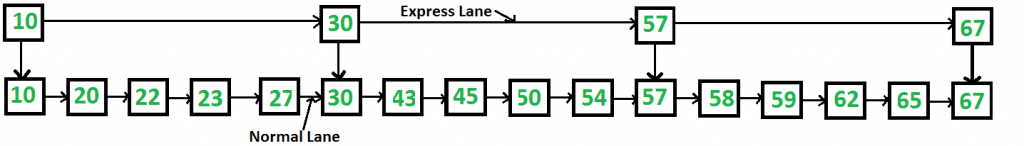# Skip List | Set 1 (Introduction)

Can we search in a sorted linked list in better than O(n) time?
The worst case search time for a sorted linked list is O(n) as we can only linearly traverse the list and cannot skip nodes while searching. For a Balanced Binary Search Tree, we skip almost half of the nodes after one comparison with root. For a sorted array, we have random access and we can apply Binary Search on arrays.

Can we augment sorted linked lists to make the search faster? The answer is Skip List. The idea is simple, we create multiple layers so that we can skip some nodes. See the following example list with 16 nodes and two layers. The upper layer works as an “express lane” which connects only main outer stations, and the lower layer works as a “normal lane” which connects every station. Suppose we want to search for 50, we start from first node of “express lane” and keep moving on “express lane” till we find a node whose next is greater than 50. Once we find such a node (30 is the node in following example) on “express lane”, we move to “normal lane” using pointer from this node, and linearly search for 50 on “normal lane”. In following example, we start from 30 on “normal lane” and with linear search, we find 50.What is the time complexity with two layers? The worst case time complexity is number of nodes on “express lane” plus number of nodes in a segment (A segment is number of “normal lane” nodes between two “express lane” nodes) of “normal lane”. So if we have n nodes on “normal lane”, √n (square root of n) nodes on “express lane” and we equally divide the “normal lane”, then there will be √n nodes in every segment of “normal lane” . √n is actually optimal division with two layers. With this arrangement, the number of nodes traversed for a search will be O(√n). Therefore, with O(√n) extra space, we are able to reduce the time complexity to O(√n).

Can we do better?
The time complexity of skip lists can be reduced further by adding more layers. In fact, the time complexity of search, insert and delete can become O(Logn) in average case with O(n) extra space. We will soon be publishing more posts on Skip Lists.